# Word Formulas

Office Apps
Word

It is a common practice to perform calculations in Excel, and then to copy the calculations into Word. A drawback to this approach is the double entry (and double update) of values.

There is another option: Word formulas.

I have created a simple table in Word 2013 with 4 columns and 5 rows and put some information in: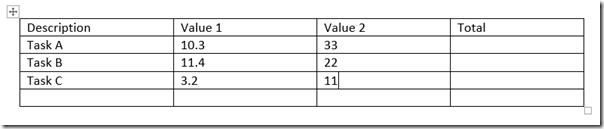It doesn’t look like much and doesn’t have any totals, so first off, I am going to make it look a little better: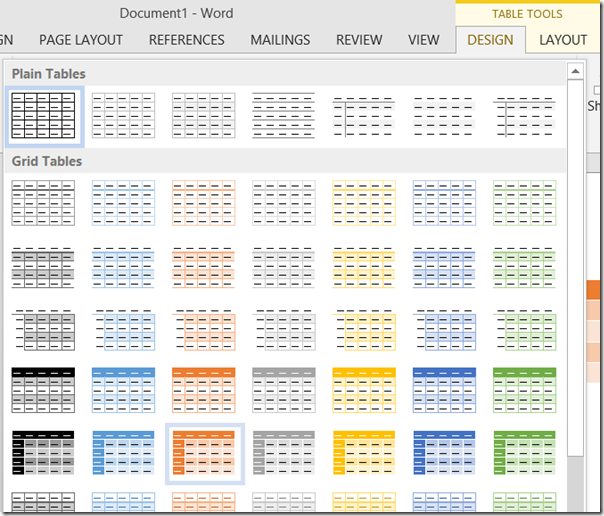I have selected the DESIGN tab and chosen an orange theme for the table so it now looks like this: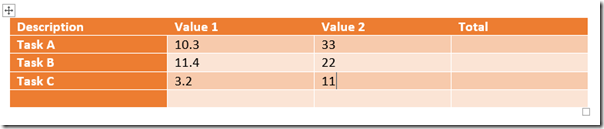Now I do not want the first column to be quite so orange, but I do want a total column on the left and a total row at the bottom.

Again, under the DESIGN tab, I have selected the Table Style Options that I want: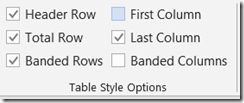And now the table looks like this: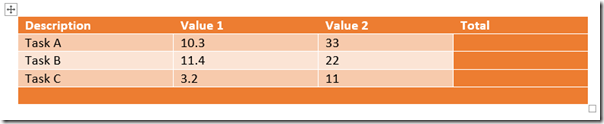Almost there, all that remains is the totals.

First off, the row totals. Placing the cursor in the total column for the first row and selecting the LAYOUT tab displays the Formula button in the ribbon: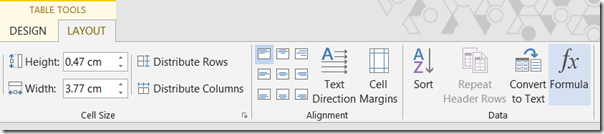This displays a dialogue box to allow a formula to be entered into the cell: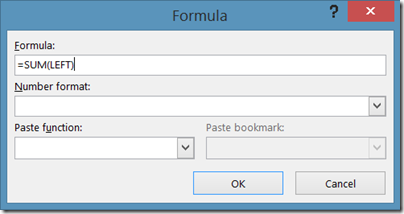The formula the is need to SUM the values to the LEFT is SUM(LEFT).

There are options for the format of the number that are similar to those in Excel, and there are other functions that can be selected fro the Paste function drop-down.

Once the value of the formula has been inserted into each of the rows, the values will be displayed.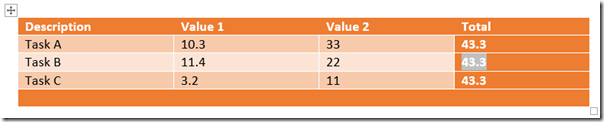But as can be seen in the image above, if you copy the formula, the previous value will be displayed. To update the values, place the cursor in the value generated from the formula and press F9 or right click and select Update Field.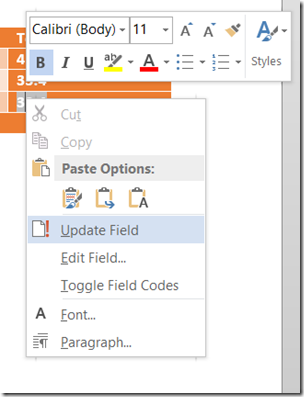To get the totals in the bottom row insert a formula with the value SUM(ABOVE)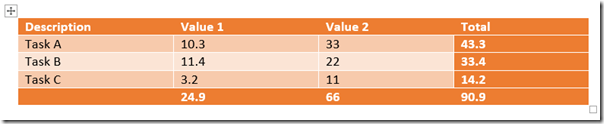Comment on this post: